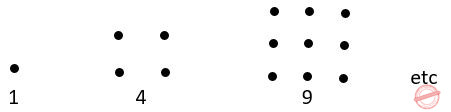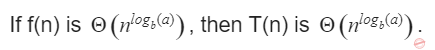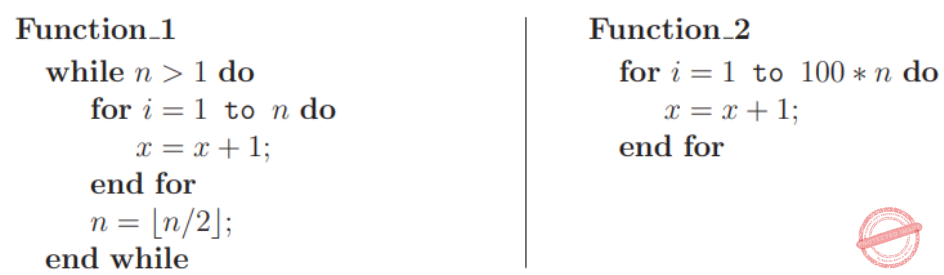## Recurrences

 Question 1

(a) Use the patterns given to prove that(You are not permitted to employ induction)(b) Use the result obtained in (a) to prove thatA Theory Explanation.
Algorithms       Recurrences       GATE 1994
 Question 2

For parameters a and b, both of which are ω(1), T(n) = T(n1/a)+1, and T(b)=1.

Then T(n) is

 A θ(loga logb n) B θ(logb loga n) C θ(log2 log2 n) D θ(logab n)
Algorithms       Recurrences       GATE 2020       Video-Explanation
Question 2 Explanation:
T(n) = T(n1/a+1, T(b) = 1
T(n) = [T(n1/a2)+1] + 1
= [T(n1/a3)+1] + 2
= [T(n1/a3)] + 3
= [T(n1/ak)] + b
= logb n = ak
= log logb n = k log a
= k= loga logb n
T(n)=1+loga logb n
T(n)=O(loga logb n)
 Question 3
For constants a ≥ 1 and b > 1, consider the following recurrence defined on the non-negative integers:Which one of the following options is correct about the recurrence T(n)?
 ABCDAlgorithms       Recurrences       GATE 2021 CS-Set-2       Video-Explanation
Question 3 Explanation:Question 4
The Lucas sequence Ln is defined by the recurrence relation:
Ln = Ln-1 + Ln-2 , for n >= 3,
with L1 = 1 and L2 = 3.
Which one of the options given is TRUE?A A B B C C D D
Algorithms       Recurrences       GATE 2023       Video-Explanation
 Question 5
Let f and g be functions of natural numbers given by f(n)=n and g(n)=n2
Which of the following statements is/are TRUE?
 A f ∈ O(g) B f ∈ Ω(g) C f ∈ o(g) D f ∈ Θ(g)
Algorithms       Recurrences       GATE 2023       Video-Explanation
 Question 6
Consider functions Function 1 and Function 2 expressed in pseudocode as follows:Let f1(n) and f2(n) denote the number of times the statement “x = x + 1” is executed in Function 1 and Function 2, respectively. Which of the following statements is/are TRUE?
 A f1(n) ε Θ(f2(n)) B f1(n) ε o(f2(n)) C f1(n) ε ω(f2(n)) D f1(n) ε O(n)
Algorithms       Recurrences       GATE 2023       Video-Explanation
 Question 7

The solution of the recurrence relation T(m) = T(3m/4) + 1 is :

 A θ(lg m) B θ(m) C θ(mlg m) D θ(lglg m)
Algorithms       Recurrences       UGC-NET CS 2018 JUNE Paper-2
Question 7 Explanation:
Using Masters Method:
a = 1, b = 4/3, k = 0, p = 0
Here, a = bk
So, T(m) = nlog a/ log b logp+1 n
T(m) = θ(log m)
 Question 8
The recurrence relation capturing the optimal execution time of the towers of Hanoi problem with n discs is:
 A T(n)=2T(n-2)+2 B T(n)=2T(n/2)+1 C T(n)=2T(n-2)+n D T(n)=2T(n-1)+1
Algorithms       Recurrences       Nielit Scientist-B IT 4-12-2016
Question 8 Explanation:
The recurrence equation for given recurrence function is
T(n) = 2T(n – 1) + 1
= 2 [2T(n – 2) + 1] + 1
= 2​ 2​ T(n – 2) + 3

= 2​ k​ T( n – k) + (2​ k​ – 1)
n – k = 1
= 2​ n-1​ T(1) + (2​ n-1​ – 1)
= 2​ n-1​ + 2​ n-1​ – 1
= 2​ n​ – 1
≌ O(2​ n​ )
There are 8 questions to complete.

Register Now